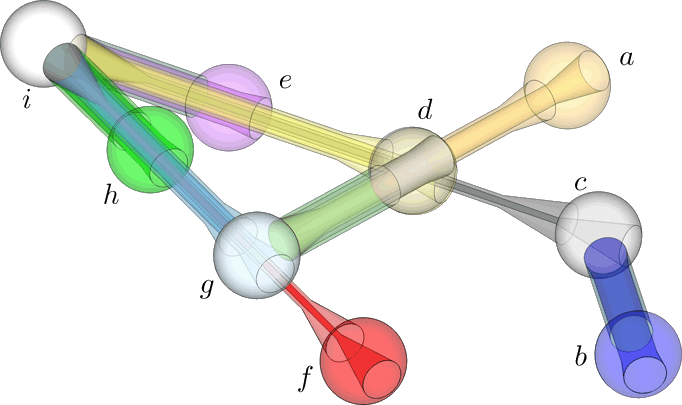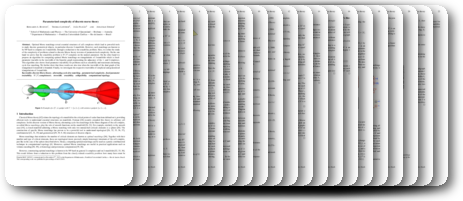## Parameterized Complexity of Discrete Morse TheoryBenjamin A. Burton, Thomas Lewiner, João Paixão, Jonathan Spreer

SoCG 2013 (29th annual Symposium on Computational geometry): pp. 127-136 (july 2013)

## Abstract:

Optimal Morse matchings reveal essential structures of cell complexes which lead to powerful tools to study discrete geometrical objects, in particular discrete 3-manifolds. However, such matchings are known to be NP-hard to compute on 3-manifolds, through a reduction to the erasability problem. Here, we refine the study of the complexity of problems related to discrete Morse theory in terms of parameterized complexity. On the one hand we prove that the erasability problem is W[P]-complete on the natural parameter. On the other hand we propose an algorithm for computing optimal Morse matchings on triangulations of 3-manifolds which is fixed-parameter tractable in the treewidth of the bipartite graph representing the adjacency of the 1- and 2-simplexes. This algorithm also shows fixed parameter tractability for problems such as erasability and maximum alternating cycle-free matching. We further show that these results are also true when the treewidth of the dual graph of the triangulated 3-manifold is bounded. Finally, we investigate the respective treewidths of simplicial and generalized triangulations of 3-manifolds.

PDF paper (2.1 MB)
PPT presentation (3.6 MB)## BibTeX:

@inproceedings{morse_complexity_socg,
author = {Benjamin A. Burton and Thomas Lewiner and João Paixão and Jonathan Spreer},
title = {Parameterized Complexity of Discrete Morse Theory},
year = {2013},
month = {july},
booktitle = {SoCG 2013 (29th annual Symposium on Computational geometry)},
pages = {127--136},
publisher = {ACM},
address = {Rio de Janeiro, Brazil},
doi = {10.1145/2462356.2462391},
url = {\url{http://thomas.lewiner.org/pdfs/morse_complexity_socg.pdf}}
}

Last modifications on July 3rd, 2013